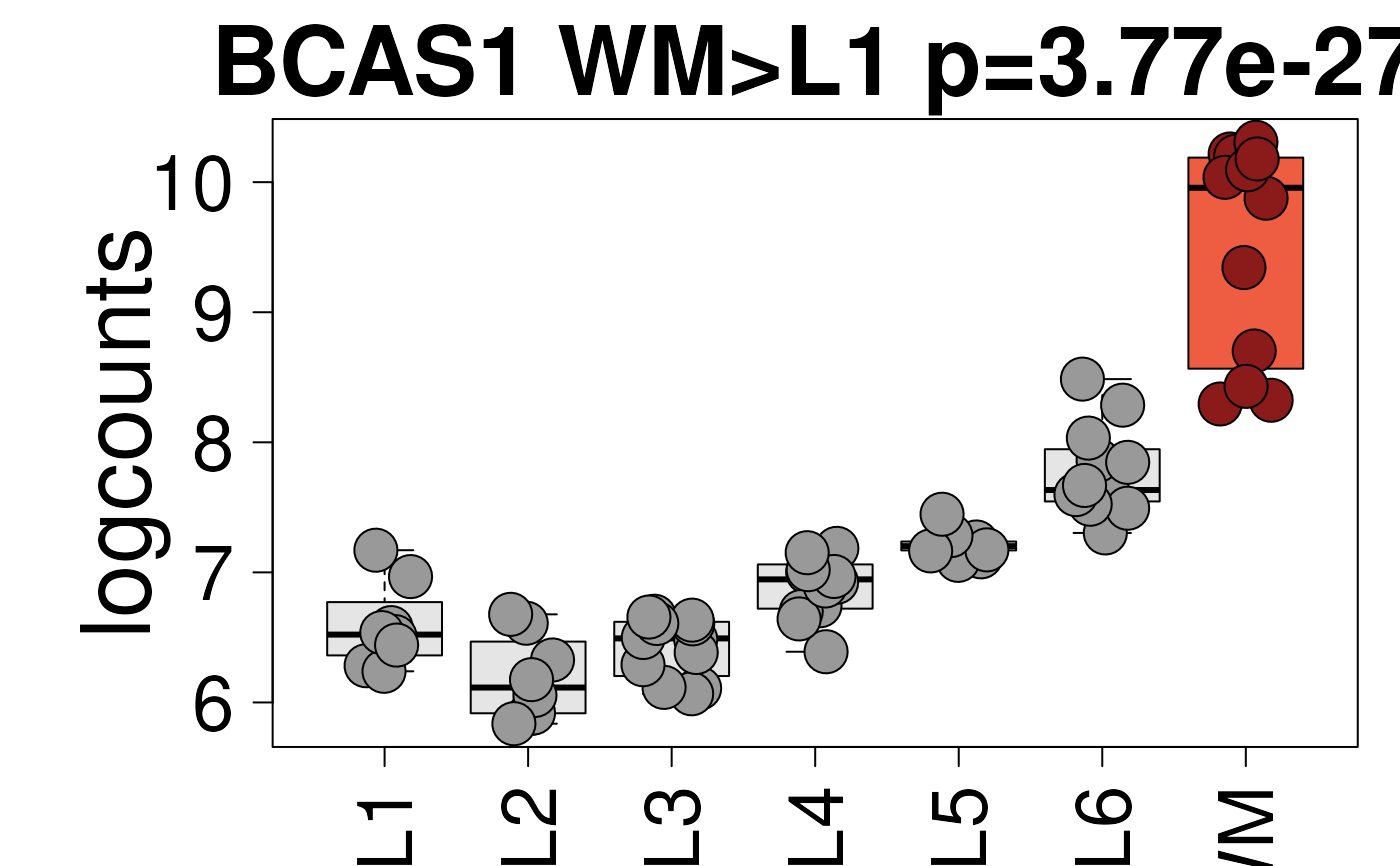This function uses the output of sig_genes_extract_all() as well as the logcounts from the layer-level (group-level) data to visualize the expression of a given gene and display the modeling results for the given gene.

layer_boxplot(
i = 1,
sig_genes = sig_genes_extract(),
short_title = TRUE,
sce_layer = fetch_data(type = "sce_layer"),
col_bkg_box = "grey80",
col_bkg_point = "grey40",
col_low_box = "violet",
col_low_point = "darkviolet",
col_high_box = "skyblue",
col_high_point = "dodgerblue4",
cex = 2,
group_var = "layer_guess_reordered_short",
assayname = "logcounts"
)

## Arguments

i

A integer(1) indicating which row of sig_genes do you want to plot.

sig_genes

The output of sig_genes_extract_all().

short_title

A logical(1) indicating whether to print a short title or not.

sce_layer

Defaults to the output of fetch_data(type = 'sce_layer'). This is a SingleCellExperiment object with the spot-level Visium data compressed via pseudo-bulking to the layer-level (group-level) resolution. See fetch_data() for more details.

col_bkg_box

Box background color for layers not used when visualizing the pairwise model results.

col_bkg_point

Similar to col_bkg_box but for the points.

col_low_box

Box background color for layer(s) with the expected lower expression based on the actual test for row i of sig_genes.

col_low_point

Similar to col_low_box but for the points.

col_high_box

Similar to col_low_box but for the expected layer(s) with higher expression.

col_high_point

Similar to col_high_box but for the points.

cex

Controls the size of the text, points and axis legends.

group_var

A character(1) specifying a colData(sce_layer) column name to use for the x-axis.

assayname

A character(1) specifying the default assay to use from assays(sce_layer).

## Value

This function creates a boxplot of the layer-level data (group-level) separated by layer and colored based on the model type from row i of sig_genes.

Other Layer modeling functions: sig_genes_extract_all(), sig_genes_extract()

## Examples


## Obtain the necessary data
if (!exists("modeling_results")) {
modeling_results <- fetch_data(type = "modeling_results")
}
if (!exists("sce_layer")) sce_layer <- fetch_data(type = "sce_layer")

## Top 2 genes from the enrichment model
sig_genes <- sig_genes_extract_all(
n = 2,
modeling_results = modeling_results,
sce_layer = sce_layer
)

## Example default boxplot
set.seed(20200206)
layer_boxplot(sig_genes = sig_genes, sce_layer = sce_layer)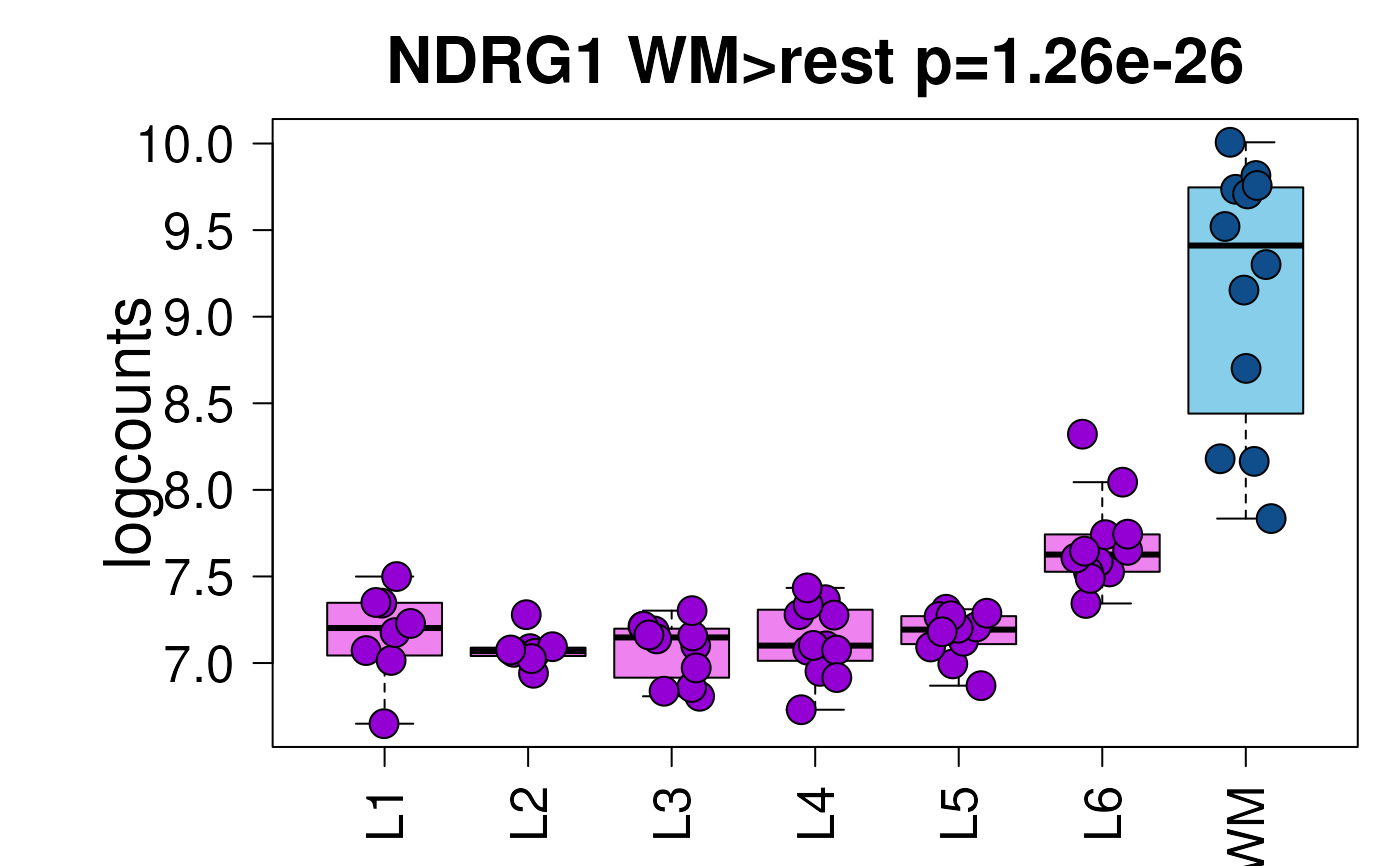## Now show the long title version
set.seed(20200206)
layer_boxplot(
sig_genes = sig_genes,
short_title = FALSE,
sce_layer = sce_layer
)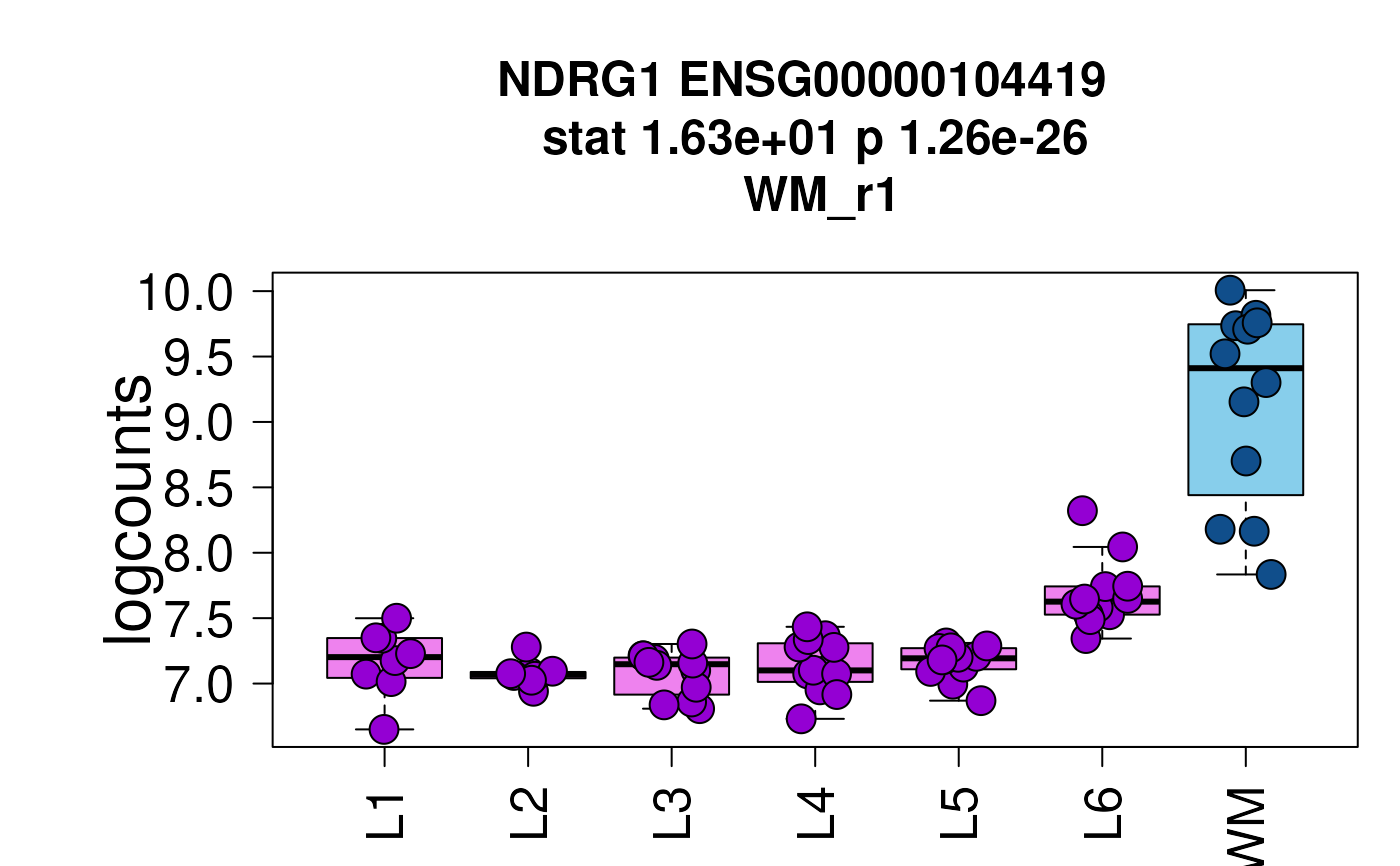set.seed(20200206)
layer_boxplot(
i = which(sig_genes$model_type == "anova"), sig_genes = sig_genes, sce_layer = sce_layer )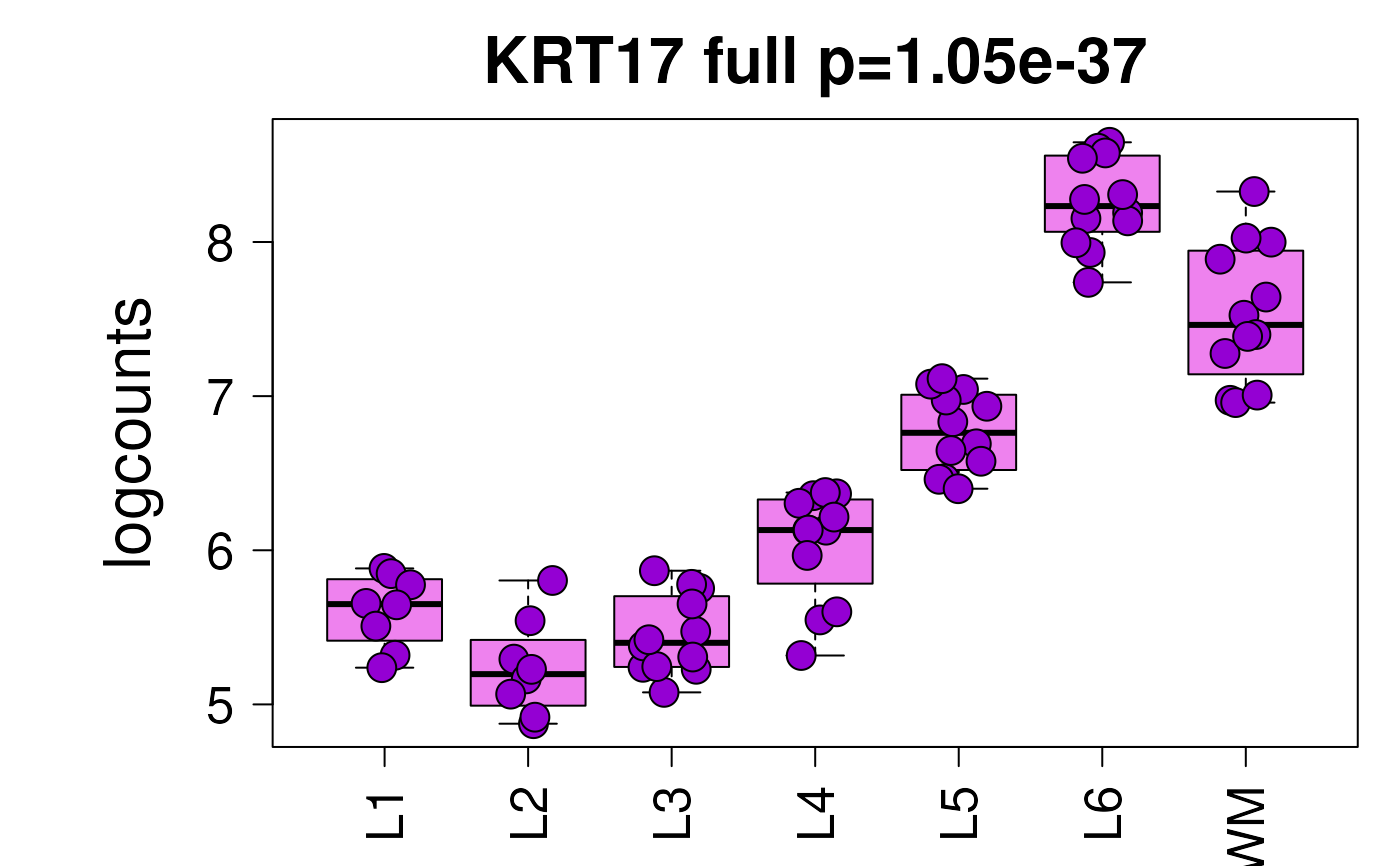set.seed(20200206) layer_boxplot( i = which(sig_genes$model_type == "pairwise"),
sig_genes = sig_genes,
sce_layer = sce_layer
)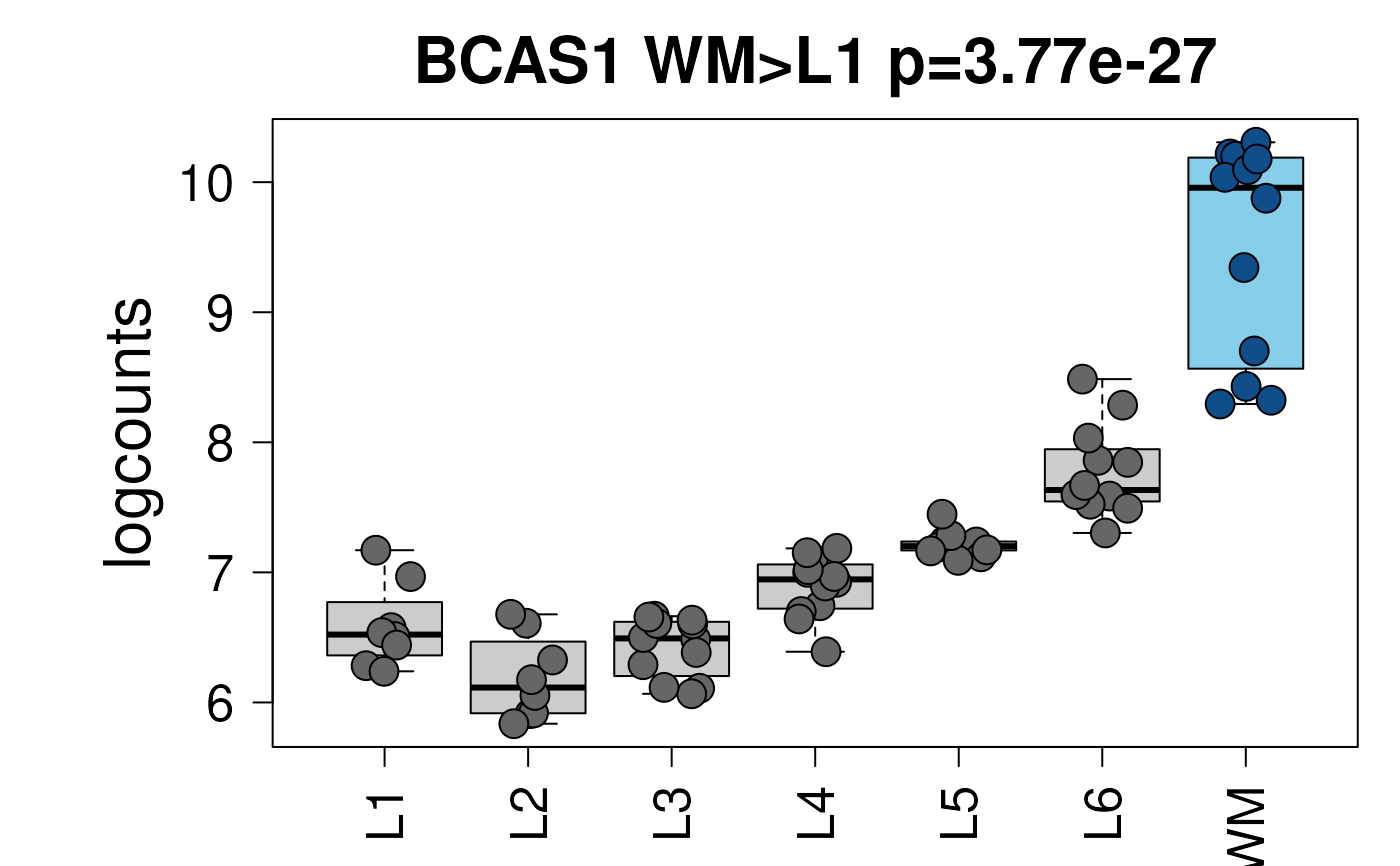## Viridis colors displayed in the shiny app
library("viridisLite")
set.seed(20200206)
layer_boxplot(
sig_genes = sig_genes,
sce_layer = sce_layer,
col_low_box = viridis(4),
col_low_point = viridis(4),
col_high_box = viridis(4),
col_high_point = viridis(4)
)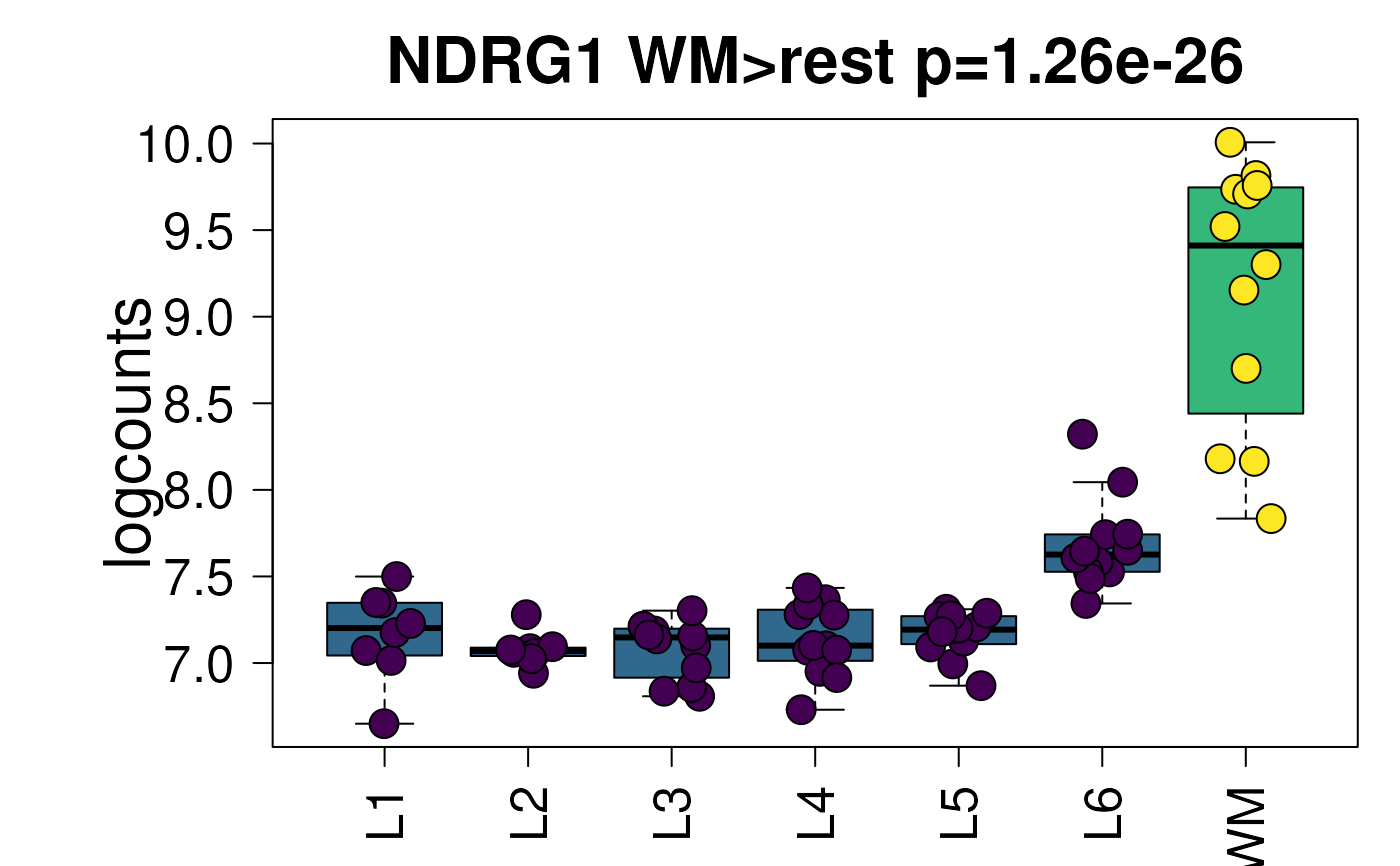## Paper colors displayed in the shiny app
set.seed(20200206)
layer_boxplot(
sig_genes = sig_genes,
sce_layer = sce_layer,
col_low_box = "palegreen3",
col_low_point = "springgreen2",
col_high_box = "darkorange2",
col_high_point = "orange1"
)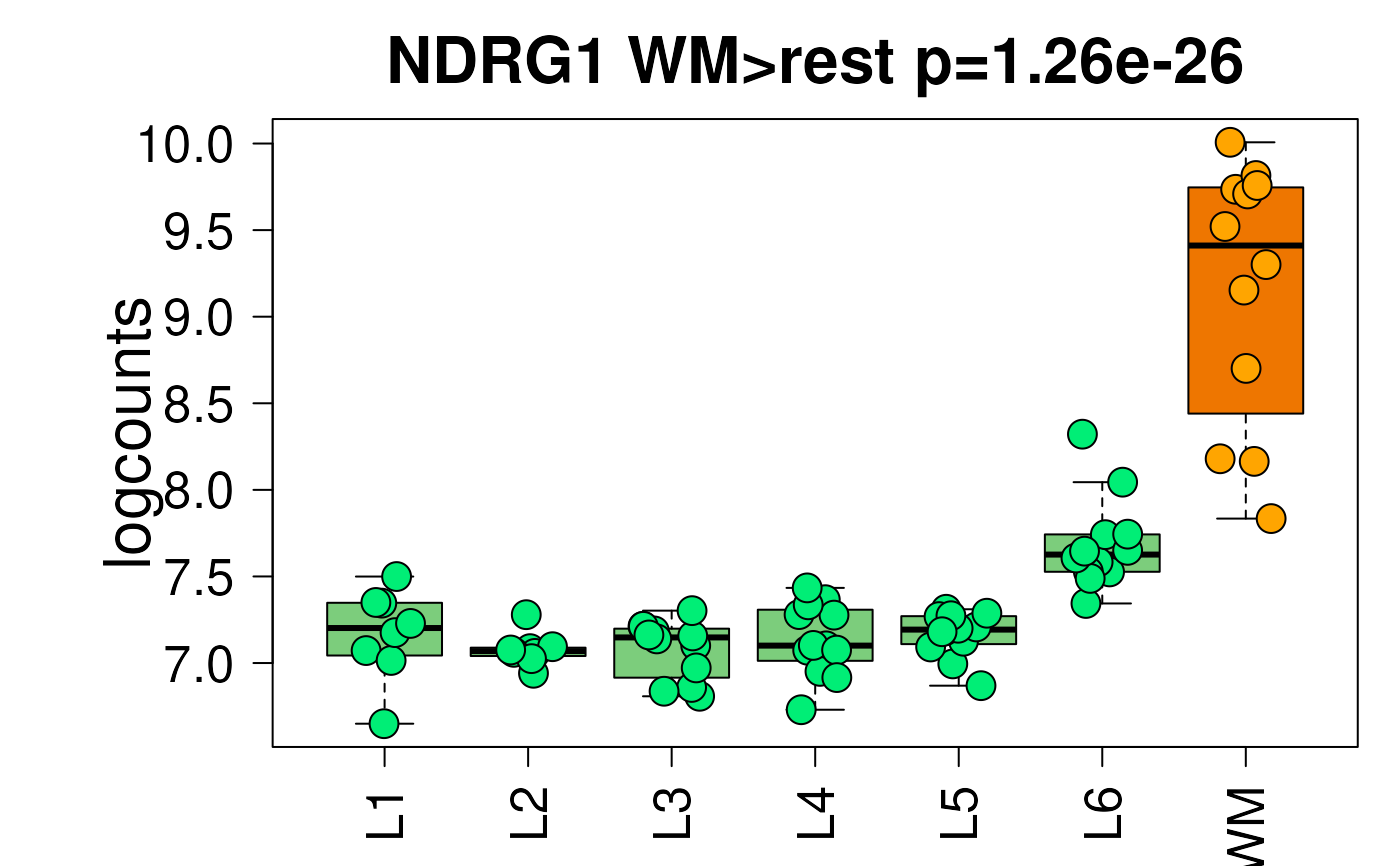## Blue/red colors displayed in the shiny app
set.seed(20200206)
layer_boxplot(
i = which(sig_genes\$model_type == "pairwise"),
sig_genes = sig_genes,
sce_layer = sce_layer,
col_bkg_box = "grey90",
col_bkg_point = "grey60",
col_low_box = "skyblue2",
col_low_point = "royalblue3",
col_high_box = "tomato2",
col_high_point = "firebrick4",
cex = 3
)